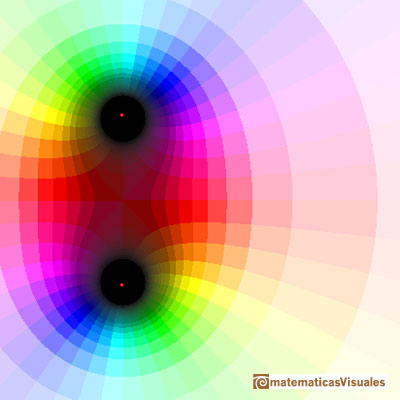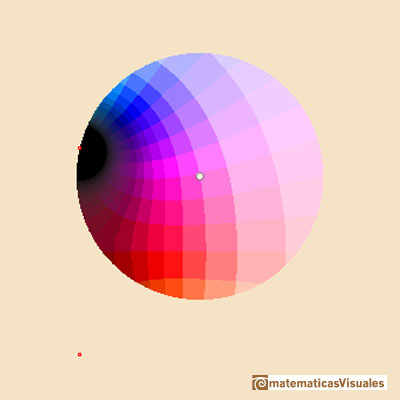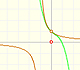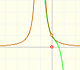We are going to study the complex functionThis complex function helps us to understand the behavior of the Taylor series of a real function that has no real singularity. This real function coincides with the complex function in the real axis:The real function has no singularities but the complex one has two singularities, in z = i and in z = -i.If we consider complex power series centered at some point, there exists a circle centered at this point such that the series converges everywhere inside the circle and diverges everywhere outside the circle. We are going to see that the radius of convergence is the distance from the center to the nearest singularity.

It is a very important fact that if this series converges at a point, then its value can be approximated by a partail sum (polynomial), and by choosing a sufficiently large degree we can make the approximation as accurate as we wish. (Tristan Needham)

For example, this is a representation of the Taylor's polynomial of degree 6:The Remainder is the difference between the function and the polynomial. A clear color, almost white, indicates a small module. As we increases the degree of the polynomial the white area approximates the circle of convergence.

This is a representation of the remainder when we consider the Taylor's polynomial of degree 6. The approximation is very accurate near the center:The approximation is better when we consider the polynomial of degree 50:If we restric our vision to the circle of convergence we can see how these polynomials approximate the function inside the circle of convergence.

This is a representation of the Taylor's polynomial of degree 50 only inside the circle of convergence:And this is a representation of the function only inside the same circle. We can see that the image below is very similar to this.Another example, when the Taylor's polynomial has only degree 15:The same polynomial but only inside the circle of convergence:And the function restricted to the same circle:Inside the circle of convergence the function and the approximation are similar but outside they are very different.

REFERENCES

Tristan Needham - Visual Complex Analysis. (pags. 64-77) - Oxford University PressThe complex exponential function is periodic. His power series converges everywhere in the complex plane.The power series of the Cosine Function converges everywhere in the complex plane.This is a continuos function and has no real singularities. However, the Taylor series approximates the function only in an interval. To understand this behavior we should consider a complex function.By increasing the degree, Taylor polynomial approximates the exponential function more and more.By increasing the degree, Taylor polynomial approximates the sine function more and more.The function is not defined for values less than -1. Taylor polynomials about the origin approximates the function between -1 and 1.The function has a singularity at -1. Taylor polynomials about the origin approximates the function between -1 and 1.The function has a singularity at -1. Taylor polynomials about the origin approximates the function between -1 and 1.This function has two real singularities at -1 and 1. Taylor polynomials approximate the function in an interval centered at the center of the series. Its radius is the distance to the nearest singularity.Examples of complex functions: polynomials, rationals and Moebius Transformations.